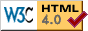Hyperbolic Tesselations Applet
alt="Your browser understands Java but can't seem to run this applet, sorry." > \n"); ?> \n"); ?> \n"); ?> \n"); ?> \n"); ?>
The following keys and mouse actions do interesting things:
(NOTE: you may have to click on the applet once before it will accept keyboard input. On some browsers (mozilla) you may have to leave and re-enter the browser window.)
```    What:
'p' - increment p (number of sides of each polygon)
'P' - decrement p
'q' - increment q (number of polygons surrounding each vertex)
'Q' - decrement q
'o' - cycle operation through the following list:
Truncate=0 (primal)
Truncate=.5
Truncate=1
Truncate=1.5
Truncate=2
Truncate=2.5
Truncate=3
Truncate=3.5
Truncate=4 (dual)
halfway between dual and runcinated
Runcinated
halfway between runcinated and primal
Omnitruncated
'O' - cycle operation backwards through above list
Right mouse - change to Omnitruncated and drag vertex around

Ctrl-'p' : toggle drawing of primal (initially on)
Ctrl-'d' : toggle drawing of dual (initially on)
Ctrl-'s' : toggle drawing of snub (initially off) (only interesting when omnitruncated)
Ctrl-Shift-'S' : switch handedness of snub
Ctrl-'o' : move vertex so that snub is uniform

Where:
Left mouse drag - change orientation
'r' - reset orientation

How:
'm' - toggle model between Poincare disk and Klein disk
'i' - increment max isometries (how much to draw) by 10
(Ctrl-'i' to increment by 1)
'I' - decrement max isometries (how much to draw) by 10
(Ctrl-Shift-'I' to decrement by 1)
0: fast (straight segment approximations)
1: fast when moving, good when stopped (default)
2: good (properly curved segments)

Debugging (stuff you don't care about):
'V' - cycle event debug level (output to java console window).
'B' - toggle double buffering
'J' - toggle random jitter of each edge (to see what's getting drawn multiple times)
'L' - cycle isometry labels style (off, label isometries, label arms)
' ' - just repaint

New bleeding edge:
Ctrl-'x': encapsulated postscript dump with black&white swapped
to file "PSDUMP.eps"
(only works when run locally, not as an applet in a browser)
```
Soon to come:
• antialiasing
• resizable
• friendly gui instead of these lame key controls
• high-quality image or postscript dumps
• filled and colored faces

#### For experts:

You can also specify a general uniform tiling, using a simplified version of the Burgiel/Conway/Goodman-Strauss (BCGS) orbifold symbol, which will be explained below. For example, here are three different tilings that all have the same list of faces around each vertex (a triangle and three hexagons):
\$s\n"); } ?>
Explanation of the notation:
• The edges surrounding the central vertex are numbered 0,1,2,... counterclockwise with edge 0 pointing to the left (unlike John Conway's drawings, which go clockwise with edge 0 pointing up).
• The first part of the symbol ( (3,6,6,6)^1 in the above examples) is the face list surrounding the vertex. The face between edges 0&1 is listed first, then 1&2, etc. The list is repeated the number of times specified in the exponent; for example (6,7,8)^2 would produce the following actual faces around a vertex: 6,7,8,6,7,8.
• The second part of the symbol is a permutation of the edges (not including replication by the exponent), the purpose of which is to describe where each edge appears in the corresponding neighbor vertex's edge list. The permutation is specified by its cycles, each of which must be of length 1 or 2. For example, the permutation (0 3)(1)(2) would mean that 0 goes to 3, 3 goes to 0, and each of 1 and 2 remains fixed. Each cycle is surrounded by either [] or () according to whether the neighbor vertex along that edge is a mirror image or a rotated image of the central vertex.
• Note that the "*" that appears in the BCGS orbifold symbol, denoting a mirror through the vertex, is not recognized; you must specify the full permutation on all the edges (not replicated by the exponent) instead.
• You can use <> in the permutation part if you like (in the orbifold symbol, this represents an edge that is split along its length by a mirror symmetry, i.e. "both [] and ()"). It is implemented as []. (This only matters if it turns out that the tiling didn't really have all the symmetries implied by the <> after all.)
You may also put <> around one or two single entries in the face list if you like (to denote that a mirror through the vertex passes through the face). These are currently ignored by the program, but you might want to use them if you want to express all the symmetry information from the BCGS orbifold symbol.
• Notes: An exponent of ^1 may be omitted. Cycles of length 1 surrounded by [] may be omitted from the permutation. (In particular, if all edge-reversing reflections are isometries of the tiling, then the permutation part may be omitted entirely.) The right brackets or parens of the cycles may be omitted; this can be convenient when experimenting (it's easier to change one character than two). For example, "(3,6,6,6)^1 (0 1)(3)" may be abbreviated as either "(3,6,6,6) (0 1[2(3" or "(3,6,6,6) (0 1(3". Underscores may be used instead of spaces throughout (this can be useful if you are embedding this notation in a URL which is not allowed to contain space characters).
 Last Modified: Mon Sep 20 04:26:54 PDT 2004 Don Hatch hatch@plunk.org